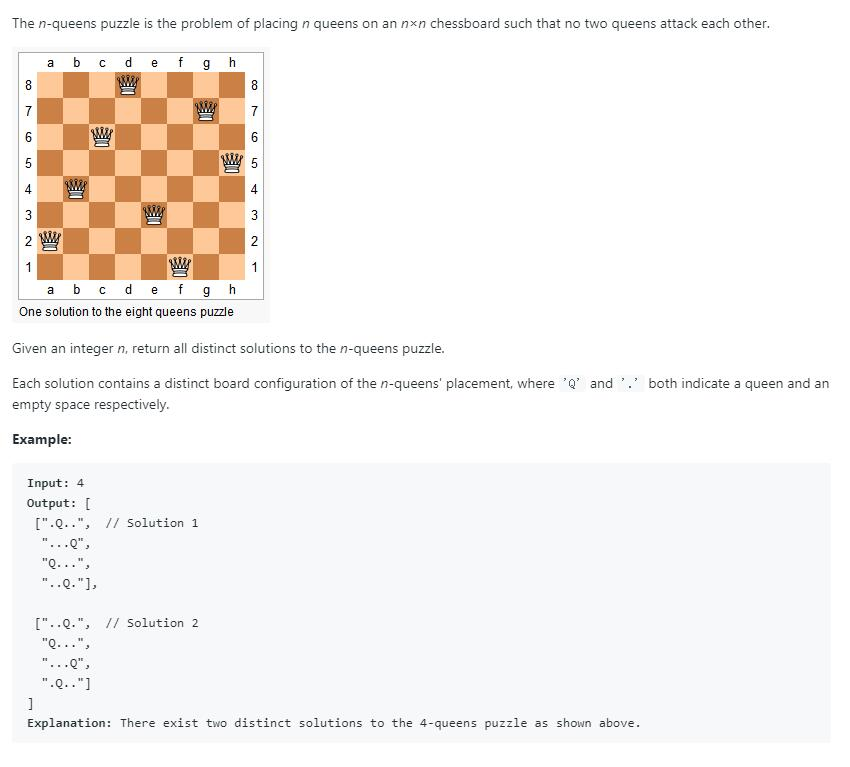# 题目描述（困难难度）# 解法一 回溯法

public List<List<String>> solveNQueens(int n) {
List<List<String>> ans = new ArrayList<>();
backtrack(new ArrayList<Integer>(), ans, n);
return ans;
}

private void backtrack(List<Integer> currentQueen, List<List<String>> ans, int n) {
// 当前皇后的个数是否等于 n 了，等于的话就加到结果中
if (currentQueen.size() == n) {
List<String> temp = new ArrayList<>();
for (int i = 0; i < n; i++) {
char[] t = new char[n];
Arrays.fill(t, '.');
t[currentQueen.get(i)] = 'Q';
}
return;
}
//尝试每一列
for (int col = 0; col < n; col++) {
//当前列是否冲突
if (!currentQueen.contains(col)) {
//判断对角线是否冲突
if (isDiagonalAttack(currentQueen, col)) {
continue;
}
//将当前列的皇后加入
//去考虑下一行的情况
backtrack(currentQueen, ans, n);
//将当前列的皇后移除，去判断下一列
//进入这一步就是两种情况，下边的行走不通了回到这里或者就是已经拿到了一个解回到这里
currentQueen.remove(currentQueen.size() - 1);
}

}

}

private boolean isDiagonalAttack(List<Integer> currentQueen, int i) {
// TODO Auto-generated method stub
int current_row = currentQueen.size();
int current_col = i;
//判断每一行的皇后的情况
for (int row = 0; row < currentQueen.size(); row++) {
//左上角的对角线和右上角的对角线，差要么相等，要么互为相反数，直接写成了绝对值
if (Math.abs(current_row - row) == Math.abs(current_col - currentQueen.get(row))) {
return true;
}
}
return false;
}